CAT  >  Vedic Mathematics: Solved Examples

# Vedic Mathematics: Solved Examples - Quantitative Aptitude (Quant) - CAT

 Table of contentsAddition and SubtractionSum of SeriesMultiplicationMethods For Multiplication By Numbers From 11 To 19.Multiplication Of Two 2 Digit NumbersMultiplication Of 2 Three-digit NumbersComparison Of FractionsPercentageUse of following tips makes the subtraction and addition operation easy and less time-consuming, in this
method we change the numbers in the easy form and then we solve accordingly. Let’s take an example to understand this method.

Example 1: Suppose we have to add 689 and 95.

We know 95 is nearer to 100, by keeping this in mind we can add 100 to 689 and subtract 5 later.
Hence 689 + 100 = 789 - 5 = 784, which is the required answer.

Take some other examples:
2. 67 + 693 = ?
It can be solved like 67 + 700 = 767 - 7 = 760.

3. 454 + 27 = ?
It can be solved like 454 + 30 = 484 - 3 = 481.

Similarly subtraction operation can be solved using these tips.
Let’s take some examples:
1. 367 - 37 = ?
It can be solved like, 367 - 40 = 327 + 3 = 330.
2. 289 - 58 = ?
It can be solved like, 289 - 60 = 229 + 2 = 231.

## Sum of Series

Sometime we have to add many numbers which are in series, i.e. they are in certain fashion.

For example,

1. Consecutive numbers:

1, 2, 3, 4, 5 etc; or 12, 13, 14...

2. Consecutive EVEN numbers:
2, 4, 6, 8, etc; or 12, 14, 16...

3. Consecutive ODD numbers:
3, 5, 7, 9, etc; or 13, 15, 17,

4. Consecutive negative numbers:
- 1, - 2, - 3, etc; or - 11, - 12, - 13......

5. Consecutive (EVEN) negative numbers:
- 2, - 4, - 6 etc; or - 12, - 14, - 16.........

6. Consecutive (ODD) negative numbers:
- 3, - 5, - 7, etc; or - 13, - 15, - 17...

For computing above type of sums we can use following formula.
S = (F + L) (N) / 2

Where:
S = Sum of all numbers
F = First number in sequence
L = Last number in sequence
N = Number of Terms in sequence

Other examples in which, we can use this formula is:
1, 2, 3 ….. (Up to 50)
3, 7, 11, 15, 19, 23
4, 9, 14, 19, 24, 29

## Multiplication

Methods For Multiplication Of Number By Multiple Of 10 (I.e. By 10, 100, 1000 Etc.)

This is quite simple just put the same number of zeroes behind the number as behind 1.
Example: 23 × 100

• Here there are two zeroes behind 1, hence by putting two zeroes behind 23. We will get the answer.
• Therefore 23 × 100 = 2300
Take another example e.g. 45 × 1000 = 45000

Methods For Multiplication Of Number By 5

• Let n is an number which is to be multiplied by 5.
i.e. n x 5 = ?
• Now n could be either even or odd.
• Now if n is even, just half the number and put zero behind that.

Example: 44 x 5
Here 44 is an even number, now half of the 44 is 22 and by putting ‘0’ it become 220. Hence answer is 220.

• Now if n is odd, subtract one from n, and half that number (i.e. n - 1) and put five behind that.
For example 47 x 5
• Here 45 is an odd number, now 47 - 1 = 46, half of this (46 / 2) is 23 and by putting ‘5’ behind that it become 235.
•  Hence, the answer is 235.

Methods For Multiplication By 25

We know that 25 = 100 / 4, hence to ease the computation, multiply the number by 100 (it is very simple just put two zeroes at the end of the number) and then divide the number by 4.

Lets take one example

• 76 x 25 = ?
• Now first multiply 76 by 100 i.e. 76 x 100 = 7600
• Now divide 7600 by 4 i.e.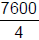= 1900, hence the answer.

Methods For Multiplication By 25
As 50 =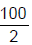, hence to ease the computation, multiply the number by 100 and then divide it by 2.
For example :

• 88 x 50 = ?
• First multiply 88 by 100, i.e. 88 x 100 = 8800.
• Now divide it by 2, i.e. 8800 by 2 i.e.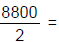4400, hence the answer.

Methods For Multiplication By 125

We know that 125 =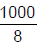, hence to ease the computation, multiply the number by 1000 (it is very simple just put three zeroes at the end of the number) and then divide the number by 8.

Lets take one example
48 x 125 = ?

• Now first multiply 48 by 1000 i.e. 48 x 1000 = 48000
• Now divide 48000 by 8 i.e.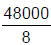= 6000, hence the answer.

## Methods For Multiplication By Numbers From 11 To 19.

Multiplication By 11

Rule:

1. Prefix a zero to the multiplicand
2. Write down the answer one figure at a time, from right to left as in any multiplication.
The figures of the answer are obtained by adding to each successive digit of the multiplicand its
right neighbour. Remember the right neighbour is the right, (i.e., the correct) neighbour to be
(1) 123 × 11 =?

Step 1: Prefix a zero to the multiplicand so that it reads 0123.

Step 2: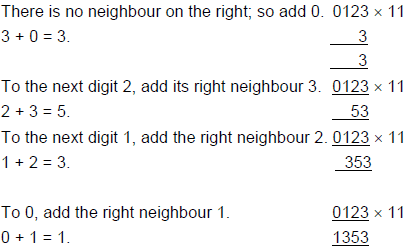Therefore, 123 × 11 = 1353 (which you can easily verify by a conventional multiplication).

Multiplication By 12
The method is exactly the same as in the case of 11 except that you double each number before

(1) 13 × 12 = ?

Step 1: Prefix a zero to the multiplicand so that it reads 013.

Step 2: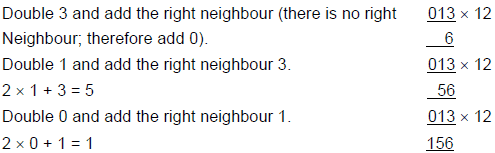Therefore, 13 × 12 = 156 (which you can again verify by a conventional multiplication).

Multiplication From 13 To 19
The reason why the rule is different for multiplication by 11 and by 12 is obviously because the right digits are different.

• The right digit, we could call the Parent Index Number (PIN). Thus in 11, the PIN is 1, and in 12 it is
2. (In 13, it is 3; in 14, it is 4 etc.) When the PIN is 1, we are simply taking each figure of the multiplication and (we could call this figure the Parent Figure - PF in short) as such and adding the right neighbour.
• When the PIN is 2, we are doubling the PF and then adding the right neighbour.
• Obviously, if the PIN is 3 (as in 3), we would treble the PF and then add the right neighbour.
• If the PIN is 4 (as in 14), we would quadruple (i.e. multiply by 4) the PF and then add the right neighbour.
• If PIN is 9 (as in 19), we would multiply the PF by 9 and then add the right neighbour, what is the advantage of the method?
We need know the tables only up to 9 and still multiply by a simple process of addition. Let us see some examples below: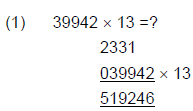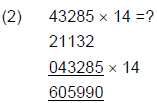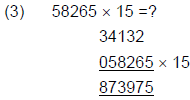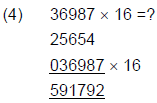## Multiplication Of Two 2 Digit Numbers

Consider the conventional multiplication of two 2-digit numbers 12 and 23 shown below: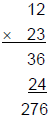It is obvious from the above that

(1) the right digit 6 of the answer is the product obtained by the "vertical" multiplication of the right digit of
the multiplicand and of the multiplier.

(2) the left digit 2 of the answer is the product obtained by the "vertical" multiplication of the left digit of the
multiplicand and of the multiplier.

(3) the middle digit 7 of the answer is the sum of 3 and 4. The 3 is the product of the left digit of the
multiplicand and the right digit of the multiplier; the 4 is the product of the right digit of the multiplicand
and the left digit of the multiplier. This means that, to obtain the middle digit, one has to multiply
"across" and add the two products (in our example 1 × 3 + 2 × 2).

The working in our above example can therefore be depicted as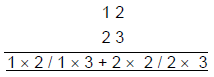and can be summarized as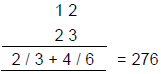When the units figure is "one" in both the numbers being multiplied, the process of multiplication is
simplified further. Consider the following multiplication: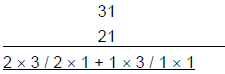You will notice that the middle digit of the answer is 2 × 1 + 1 × 3 i.e. (2 + 3) × 1. So, instead of multiplying
"across" for the middle term, you could simply add the tens digit of the two numbers.
Therefore, 31 × 21 = 6 / (2 + 3) / 1 = 651.

Similarly, in 81 × 91, you could obtain the middle term as 17 by merely adding 8 and 9.

Like wise, when the tens figure is "one" in both the numbers being multiplied, you could obtain the middle
term by simply adding the units digit of the two numbers. For instance, the middle term in 12 × 17 is 2 + 7, i.e.
9, in 8 × 12 it is 8 + 2 i.e. 10 etc.

If the units figure or the tens figure is the same in the two numbers, the process of multiplication could be
simplified as shown in the following examples:

1.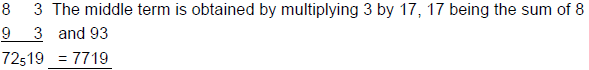2.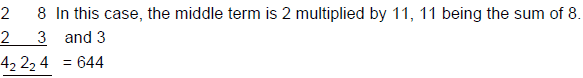Here's a rather complicated trick for calculating any two 2-digit numbers:
Note:
To do this trick, you need to know the squares of numbers up to 100 in advance.

1. Calculate the average of the two numbers.

2. Square your answer (here's where you need to know the squares of numbers through 100).

3. Subtract the largest of your original two numbers from the average.

Example: 27 × 15
First, calculate the average (42 / 2 = 21)
Then square it (212 = 441)
Now subtract largest number from the average (27 - 21 = 6)
Subtract the square from the first square (441 - 36 = 405). And there's your answer: 405
This works every time, but as the average and differences can end in .5, you have to know these
squares as well.
By the way, this trick works because (x + y)(x - y) = x × x - y × y

## Multiplication Of 2 Three-digit Numbers

Let us consider the multiplicand to be ABC and the multiplier to be DEF, as shown below: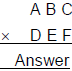1. The extreme right digit of the answer is obtained (as before) by vertical multiplication as C × F.

2. The extreme left digit is also obtained (as before) by vertical multiplication as A × B.

3. The "middle" digits are obtained (as before) by multiplying across. Progressing one step at a time to the

left, the "middle" digits are successively

B × F + C × E

A × F + C × D + B × E

A × E + B × D

The process is set out in detail for following examples below.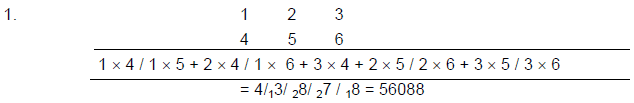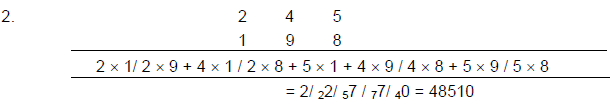### Multiplication Of Numbers Of Different Lengths

In the examples we saw above, both the multiplicand and the multiplier contained the same number of digits.

But what if the two numbers were to contain a different number of digits; for instance, how would we multiply 286 and 78?

Obviously we could prefix a zero to 78 (so that it becomes 078, a 3 digit number) and proceed as in any multiplication of two 3 digit numbers.

The following examples will clarify the procedure: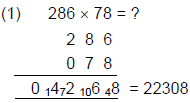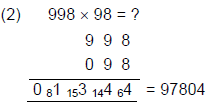### To Multiply Any Two Numbers A And B Close To A Power Of 10

a. Take as base for the calculations that power of 10 which is nearest to the numbers to be multiplied.

b. Put the two numbers A and B above and below on the left hand side.

c. Subtract each of them from the base (nearest power of 10) and write down the remainders r1 and r2 on
the right hand side either with a connecting minus sign between A & r1 and B & r2 if the numbers A and
B are less than the closest power of 10. Otherwise, use a connecting plus sign between the numbers
and the remainders.

d. The final answer will have two parts. One on the left hand side and the other on the right hand side. The
right hand side is the multiplication of the two remainders and the left hand side is either the difference
of A and r2 or B and r1 if the numbers are less than the closest power of 10. Otherwise, it is the sum of
A and ror B and r1

Few examples, which make the procedure clear, are:

Multiplication of 9 and 7
The closest base to the two numbers in this case is 10
Therefore

9 - 1 (The remainder after subtracting the number from 10)
7 - 3 (The remainder after subtracting the number from 10)

The right hand side of the answer will be 1 × 3 = 3
The left hand side can be computed either by subtracting 3 from 9 or 1 from 7 which is 6. Therefore, the
Numbers, which are close to 100
The closest base in this case will be 100
Therefore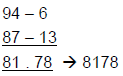Here, 6 in the first row is the difference 100 and 94 and the 13 in the second row is the difference between 100 and 87. The right hand side of the answer is obtained by the multiplication of 6 and 13 which is 78 and the left hand side is obtained by the difference between either 87 and 6 or 94 and 13, both of which give the answer 81.

Numbers which are greater than the closest power of 10
Find the product of 108 and 112
The closest base is 100 in this case as well
Therefore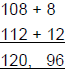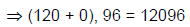The procedure is the same with only difference being that instead of subtracting the remainder of one number from the other number, we add in this case as the number were marginally larger than the nearest power of 10. When the number of digits of the product of the remainders is greater than the power of 10 closest to the two numbers
E.g.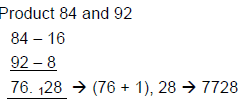As the product of 16 and 8 is 128 which is a three digit number as against 2 being the power of 10 in100, we carry forward the digits on the left more than 2 digits (in this case) and add to 76, the left hand side of the answer.
E.g. When one of the number is lesser than the closest power of 10 and the other greater than the
closest power of 10.

Product of 88 and 106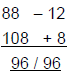The operation is similar, excepting that as the right hand side of the answer is obtained by the multiplication of a positive and a negative number the answer has to be subtracted from 100 by reducing the left hand side number by 1. So the answer is 9504.

Multiplication of numbers which are not close to the nearest power of 10
Let us take the case of multiplication of 41 by 43. Going by the earlier method we have the nearest power of 10 as 100 or 10. In the former case, The remainders are 59 and 57, multiplication of which will be as tedious as the multiplication of these two numbers. In the latter case, The remainders will be 31 and 33 which will be equally difficult Therefore, we need to look at an alternative method. In this case, we can take 50, which is a sub multiple of 100 or a multiple of 10 and proceed

Method 1: Take 50 as the base which is half of 100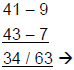since 50 = 100/2. We divide the left hand side number also by 2 while retaining the right hand
side. Therefore the answer will be 1763.

Method 2: We can start off instead of using 50 as the base, we can use 40 as the base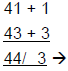and since 40 is 4 times 10, we multiply 44 by 4 to yield by 176 and join the right hand side to
yield 1763 - the same answer.

MULTIPLICATION OF ANY THREE DIGIT NUMBER WITH 101
For example
349 × 101
Add 3 and 9. This is 12. 2 is middle digit of answer, 1 is carry forward.
Take first two digits i.e. 34. Add carry forward, 1 to it. This is 35. These are first two digits of answer.
Take last two digits of number, 49. 49 is last two digits of answer.
Lets take few more examples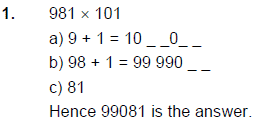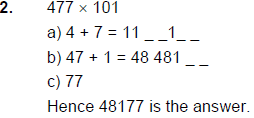MULTIPLY THREE DIGIT NUMBER WITH 7 × 11 × 13
Simply write the number twice.
e.g. 346 × 7 × 11 × 13 = 346346
845 × 7 × 11 × 13 = 845845

PRODUCT OF NOS. (ENDING WITH 5) WHICH DIFFER BY 10
E.g. 45 × 35
Step 1: First of all write 75 in the last two places of the product 45 × 35 = __75
Step 2: Then multiply 4 with 3 and add the smallest of these to the product i.e. (4 × 3 + 3) = 15
Step 3: Now Place these two digits before 75 to get our required product i.e. 45 × 35 = 1575

Product of two complementary numbers

What are they?

Before we begin the discussion on how to calculate the product, we will first define what we mean by
complementary numbers.
Complementary numbers are two numbers that add up to a power of 10. For example, 2 and 8 are
complementary, because they add up to 10. Also 22 and 78 are complementary, because they add up to 100,
and so on.

Numbers that add up to 10

Almost everyone would be expected to calculate 2 × 8 (or 2 x 8) through the memorisation of the multiplication
table. A less well known fact is that 2 x 8 equals the difference of the square of 5 (average of two and eight)
and the square of 3 (= 8 - 5 = 5 - 2), i.e. 2 × 8 = 5 × 5 - 3 × 3 = 16.

Numbers that add up to 100, 1000, 10000 etc.
To calculate the product of 42 × 58, we make use of the relationship described in the previous paragraph:
42 × 58 = (50 - 8) × (50 + 8) = 50 × 50 - 8 × 8 = 2500 - 64 = 2436.
In other words, to multiply two numbers N1 and N2, we just have to square the average number N, (= 5, 50,
500...) and subtract from it the square of the difference (N - N1).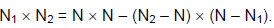Examples:
45 × 55 = 50 × 50 - 5 × 5 = 2500 - 25 = 2475.
4899 × 5101 = 5000 × 5000 - 101 × 101 = 25,000,000 - 10201 = 24,989,799

Product of two partially complementary numbers

What are partially complementary numbers?

We have seen that complementary numbers add up to a power of 10. Partially complementary numbers have
their first digits common (the root), and their last digits (suffix) add up to a power of 10. For example, 43 and
57 are complementary, because 43 and 57 add up to 100, a power of 10. On the other hand, 343 and 357 are
partially complementary, the root is 3 and the suffices are 43 and 57.

Suffices that add up to 10
To calculate the product, you would have to calculate product of the root and the next number, and append
the product of the suffices.

To multiply 53 by 57, we identify that the root is 5 and the suffices are 3 and 7. The product of the root and the
next number is therefore 5 × (5 + 1) = 30. Appending the product of 3 and 7 (= 21) to 30 gives 3021.
Thus 53 × 57 = 3021.

Note that this is almost the same procedure as the calculation of squares of numbers ending in 5. Instead of
appending 25, we append the product of the suffices.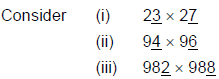In all of them, the units figures together total 10 [3 + 7] in (i), 4 + 6 in (ii) and 2 + 8 in (iii)], also the other figures of the multiplicand and the multiplier are the same [2 in (i) 9 in (ii) and 98 in (iii)].

In such cases, a special method can be used. But before we see the new method, let us consider the
multiplication of 23 by 27 by the method we had learnt. 23 × 27 would give us the answer as 621. Do you
notice anything special about the answer? The right part is the product of the unit figures 3 and 7 of the 2
numbers and the left part 6 of the answer is the product of 2 (the tens figure) and the next higher number 3.

This gives us the special rule:
(1) To obtain the right part of the answer, multiply the units (i.e. of the extreme right) digit of the 2 numbers.
(2) To obtain the left part of the answer, multiply the other (i.e. the left), digit/s by one more than
itself/themselves. For example, if the left digit/s of the 2 numbers is/are

3, multiply 3 by (3 + 1) i.e. by 4
5, multiply 5 by 6
6, multiply 6 by 7
99, multiply 99 by 100
100, multiply 100 by 101
888, multiply 888 by 889 etc

The only thing you have to be careful about is to ensure that the right part of the answer always has 2 digits.
For example in 29 × 21, the left part of the answer is 2 (2 + 1) and the right part is 9 × 1 i.e. 9; however the
right part will be written as 09 and not as 9 because the right part has to contain 2 digits. Therefore, 29 × 21 =
609.

Suffices that add up to 100, 1000, 10000 etc.

The same steps will be followed. Multiply the root by the next number. Append the product of the suffices.
For example, for 1545 × 1555, the root is 15, suffices are 45 and 55. Thus the product of the root and the next
number is 15 × (15 + 1) = 240. To this, we append the product of the suffices, 45 × 55 = 2475. The suffices
here are two digits long, and their product must therefore occupy four digits. Thus 1545 × 1555 = 2402475.

MULTIPLICATION OF NUMBERS WHICH ARE CLOSE TO EACH OTHER
24 × 26 = ?
28 × 32 = ?
87 × 93 = ?
In this shortcut we use the application of algebra. we know a2 - b2 = (a + b) (a - b)
Now as 24 × 26 can be written like (25 + 1) × (25 - 1),
Now using above formula it is equal to 252 - 12 = 625 - 1 = 624, which is the required answer.
Similarly
87 × 93 = (90 + 3) x (90 - 3) = 902 - 32 = 8100 - 9 = 8091
28 × 32 = (30 + 2) × (30 - 2) = 302 - 22 = 900 - 4 = 896

SQUARES OF NUMBERS
It is often useful to know the squares of numbers without a calculator. For example, you have to paint the
ceiling of a room of 12'-6" × 12'-6". How much paint would you buy if a quart covers 8 square metres? You
would reason as follows:

A quart covers 8 square metres = 80 sq.ft.

12.5 x 12.5 = 156.25, therefore you'll need 2 quarts of paint to cover 160 sq.ft.

In general, it is quite possible that an average person would be able to give the squares of integers up to 100
without a calculator. Some of the numbers are easily memorized, and the others can be obtained by certain
'tricks'. This article provides some tools to memorize or calculate the square of some numbers.

Numbers Method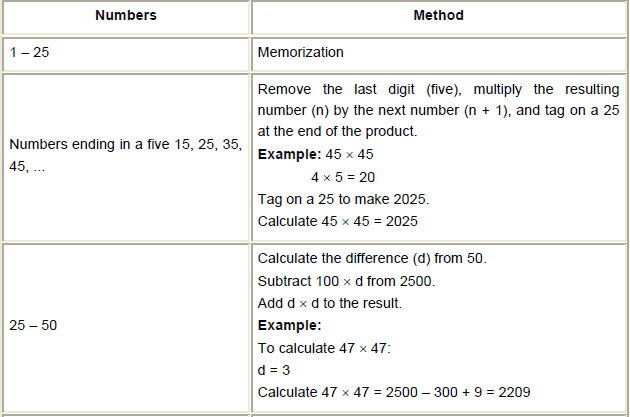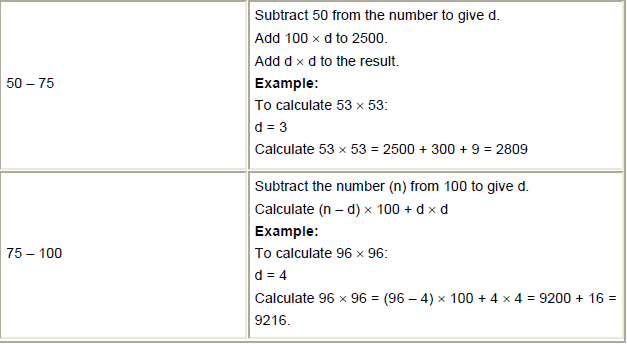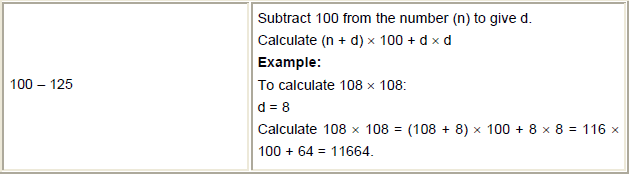Some other Methods of Squaring

I know, I know, 11 and 12 shouldn't pose any problem but anything higher than 13 was never a
flashcard...
Square the ones digit. This becomes your last digit.
1. Multiply the ones digit by 2. This becomes your second digit.
2. Retain the tens digit (i.e., 1) and append the above results.
Take 172, for example.
72 = 49. The 9 is your last digit. Carry the 4 to the left.
7 x 2 = 14. The 4 (plus the 4 remaining from the first step) is your second digit, 8. As before carry the 1
to the left.
Retain the 1 from "17", add to it the 1 remaining from the previous step, and you get 289.
Interesting but probably not as quick as simply multiplying the numbers.

2. General Method of squaring numbers 51 to 100.
1. Subtract the number you want to square from 100. (100 - x)
2. Take this difference and subtract it from the number you want to square. (x - (100 - x)). This is the
3. Square the difference, the answer from step 1. This is the last 2 digits of your answer.
Take 962, for example.
100 - 96 = 4.
96 - 4 = 92. You're halfway there. 92__.
Try 882.
100 - 88 = 12
88 - 12 = 76. 76__.
122 = 144. What now? 882 isn't 76144! 922 was only 9216.
OK enough suspense. Just carry the 1 over the 6.
_144
76__
7,744

If you like that, well check this out for numbers 50-59:
It's a simple rule: Square the tens digit and add to that the ones digit of your original number. That's your first
two numbers. Second, square your ones digit. That's your last two numbers.

Get it? Try it with 54. It should make sense.
1. 52 + 4 = 29. That's our first two numbers.
2. 42 = 16. That's our last two numbers. Thus, the answer 2916.

Another Method for Squaring numbers form 51 to 59.
There is one general formula

(5x)2 = (25 + x)/x2
Where x is a digit in units place
Note: Slash here does not represent division. It is just to differentiate between the two value. x2 should be a
two digit no. If it is a single digit no, make it a two digit no by placing a prefix 0 before x2
E.g. 32 = 9, Make it of two digits i.e. 09
Let us calculate the squares of 54
In this case our x is 4
(54)2 = (25 + 4)/42 = 29/16 = 2916 is our answer

What about numbers greater than 100?
Here are the steps and an example.
1. Subtract 100 from the number you want to square (x - 100).
2. Take the difference and add it to the number you want to square. (x + (x - 100)). This is the first 3 digits of
3. Square the difference, the answer from step 1. This is the last 2 digits of your answer.

Example: 1122
112 - 100 = 12
112 + 12 = 124. 124__.
122 = 144. Carry the 1 over the 4.
__144
124__
12,544

Use the same rules above. Here are 2 examples:
9962
1000 - 996 = 4
996 - 4 = 992. 992___ First 3 digits.
42 = 16. Last 3 digits.

Make sense?
10072
1007 - 1000 = 7
1007 + 7 = 1014. 1014___ First 4 digits.
72 = 49. Last 3 digits.

Another Method for squaring any two digit number
"a" is a digit in tens place. It is replaced by digits from 1 - 9.
Procedures: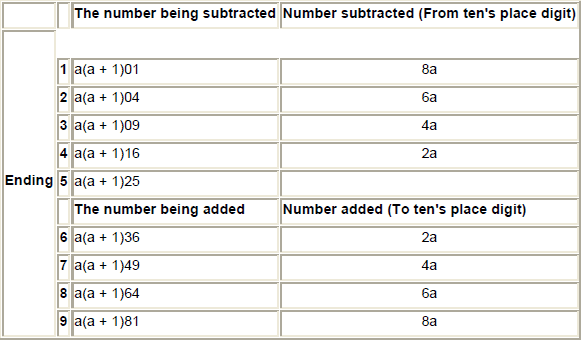Note: The numbers that are stacked to a(a + 1) are easily found by squaring the digit in the ones place. a1,
(12) then 01 is the number that will stack. a2, (22) then 04 is the number that will stack. a3, (32) then 09 is the
number that will stack. a4, (42) then 16 (Not 016) is the number that will stack. a5, 25; a6, 36; and so on.. up
to a9 in which 81 will be the number to stack on a(a + 1).
Example: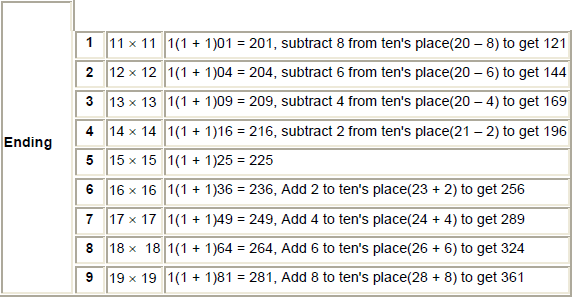How do we remember what number to add or subtract ?
If the ending digit of your two digit number is between 1 - 4, the operation is subtraction. To know what
number to subtract from the ten’s digit, add the digits in one’s place and subtract the result from ten.
[Don't forget to multiply the last result by "a"].

If the ending digit is between 6 - 9, the operation is addition. To know what number to add to the ten’s digit,
add the digits in one’s place and subtract ten from the result. [Don't forget to multiply the last result by
"a"]
Remember, use this method only for the square of two digit numbers.

SQUARING NUMBERS ENDING IN 5
An obvious extension of the above method will be in finding the square of numbers ending in 5. Consider 15 ×
15. Here the sum of the units digit of the 2 numbers is the same. Hence, the above method will apply. Infact it
will apply in squaring any number ending in 5. Since in all these cases the right digit of the multiplicand and
the multiplier is 5, the right part of the answer is always 25 and therefore, we can mechanically set down 25 as
the right part of the answer without any calculation - all that is needed is to find out the left part of the answer
and this done exactly as in the previous section.
152 = 225

252 = 625

8752 = 765625

89952 = 80910025

SQUARING NUMBERS ONLY ONES
(11)2 = ?
(111)2 = ?
(1111)2 = ?

It is very simple just count the number of ‘1’ in the question and write the natural numbers up to that number
in ascending order then in descending order up to 1. So the answer for the above questions are
(11)2 = 121
(111)= 12321
(1111)= 1234321

SQUARING NUMBERS ONLY NINES
(99)2 = ?
(999)2 = ?
(9999)2 = ?
In this method, count the number of nines in the question.
Let there are n number of nines. Then square of the number is
(n - 1) nines | 8 | (n - 1) zeroes | 1
(99)2 = two nines i.e. n = 2, hence the answer is ⇒ (2 - 1) nines | 8 | (2 - 1) zeroes | 1 = 9801
(999)= here n = 3, hence the answer is ⇒ two nines | 8 | two zeroes | 1 = 998001
(9999)2 = here n = 4, hence the answer is ⇒ 99980001

SQUARING OF NUMBER BETWEEN 101 AND 199
For example (119)2 = ?
a) Add last two digit of number to the number 119 + 19 = 138
b) Square last two digits 19 × 19 = 361
Take last two digits, 61 as last two digit of answer. Rest of the numbers are carry forwards.
c) Add carry forward, 3 to 138. 138 + 3 = 141
These are first three digits of answer.

Take few more examples.
1. (145)2 = ?
a) 145 + 45 = 190
b) 45 × 45 = 2025
c) 190 + 20 = 210

2. 106 × 106
a) 106 + 06 = 112
b) 06 × 06 = 36
c) 112 + 0 = 112

CUBE ROOTS OF EXACT CUBES

(1) The lowest cubes, i.e. the cubes of the first nine natural numbers are 1, 8, 27, 64, 125, 216, 343, 512
and 729.

(2) Thus, they all have their own distinct endings; and there is no possibility of overlapping or doubt as in
the case of squares.

(3) Therefore, the last digit of the cube root of an exact cube is obvious: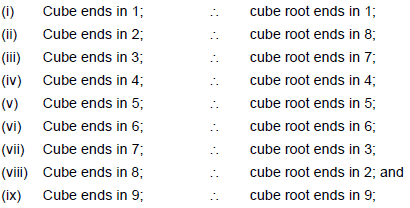(4) In other words,
(i) 1, 4, 5, 6, 9 and 0 repeat themselves in the cube-ending; and
(ii) 2, 3, 7 and 8 have an inter-play of complements from 10.

(5) The number of digits in a cube root is the same as the number of 3-digit groups in the original cube
including a single digit or a double-digit group if there is any:

(6) The first digit of the cube-root will always be obvious from the first group in the cube.

(7) Thus, the number of digits, the first digit and the last digit of the cube root of an exact cube are the data
with which we start, when we begin the work of extracting the cube root of an exact cube.

To instantly determine the cube root, follow these easy steps:
Example: Find the cube root of 438976.
1. Drop the last three digits and find the largest cube contained in 438. This is 73 = 343, so the ten’s-digit
is 7. (This is why you had to memorize the cubes of the digits 1 through 9).
2. Now go back to the last three digits. Look at the last digit, 6. That's the same ending as 63, so your
unit’s digit is 6. So the cube root of 438976 is 76.

Another example:
How do you instantly determine the cube root of 79507?
1. Drop the last three digits and find the largest cube in 79. This is 43 = 64, so the ten’s-digit of the cube
root is 4.
2. Now go back to the last three digits. Look at the last digit, 7. That has the same ending as 3-cubed. So
the unit’s-digit of your cube root is 3.
Therefore, the cube root of 79507 is 43.

APPROXIMATIONS OF SQUARE ROOTS TO TWO DECIMAL PLACES
If you have to calculate the square root of 90 fairly accurately, how do you do this quickly? Follow this thumb
rule:
(1) Find the integer whose square is nearest to 90.
(We can take 9, as 92 = 81 is close to 90)

(2) Subtract the square of this root from the number, i.e., 90.
This means 90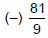(3) Divide the resulting number by twice the value of the square root (i.e., 9)
or,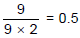This is the correction to be made to the square root approximated earlier.
Add this value to the root estimated and get the approximate value of the square root, i.e. 9 + 0.5 = 9.5.
Therefore, 9.5 is the approximate square root of 90.

Another example:
(1) Square root of 250
Nearest square is 256
Estimated square root is 16
250 minus 256 = - 6
Divide this by 2 times 16 =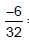= - 0.1875
Add to 16 = 16 - 0.1875 = 15.8125

Another method (Clay’s Method) for finding out approximate square root
If you're good with long division, here's a quick way to find pretty accurate square roots without the aid of a
calculator. Let's try 24.6.
1. Make a guess. It can be a very bad guess. It doesn't matter. You can even guess 1. Let's try 5 since 52
is 25, which is pretty close to 24.6.
2. Divide 24.6 by 5. 24.6 / 5 = 4.92
3. Now, comes the trick: Pick a new guess between 5 and 4.92 and divide it into 24.6 again. Let's try
4.95. 24.6 / 4.95 = 4.96. 4.96 is pretty close to 4.9598 which is the actual square root of 24.6.
4. Repeat steps 2 and 3 to any desired level of accuracy. The further you go, the harder the long division
becomes. But the first few cycles yield a pretty close answer.
The reason this works is because n*n = 24.6 and n = 24.6 / n. Therefore, the real square root will
always be somewhere between 24.6 / n and n.

Another method (Newton’s method) to find the square root of a number.
Let's try to calculate the square root of 24.6. As Clay did, the initial estimate is 5. So N = 24.6, and
E = 5.
The Newton's method says a better estimate, E1 is E1 = E + (N - E × E)/(2E).
= 5 + (24.6 - 5 × 5)/(2 × 5)
= 5 - 0.4 / 10
= 4.96 (error = 0.0033%)
This can easily be handled using mental arithmetic, and is pretty close to the real value of 4.9598. Continuing
to use mental arithmetic (see Clay's article on multiplication tricks), we can mentally calculate
4.96 × 4.96 = 5 × 5 - 2 × 0.04 × 5 + 0.0016 = 24.6016.
This time the residue is 0.0016, divided by 2 × 4.96 = 9.92 gives the next estimate,
E2 = E1 + (N - E1 × E1) / (2 × E1)
= 4.96 - .0016/9.92¸
Now, we can look at how we can handle the division. The approximation 0.0016/10 = 0.00016 is a very close.
In fact, it gives E2 = 4.95984, the error is 0.0000261%!
However, if you want to push your mental arithmetic, the division mentally is possible! You notice that dividing
by 9.92 instead of 10 gives 0.8% more to the result, which we can simply add to 0.00016 + 0.00000128 =
0.00016128
Therefore
E2 = E1 - 0.00016128 = 4.95983872, compared to the actual value of 4.959838707 (error of 0.0000003%). I
think we fare better than calculators displaying up to 8 digits, using just our brains, not even pencil and paper!

## Comparison Of Fractions

We know that, if denominator of the all fractions is same, then fraction having bigger numerator is bigger in value and having smaller numerator is smaller in value.
Let’s take one example.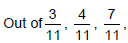the first fraction is the smallest and the last one is the biggest.
Also if the numerator of all fractions is same, then the fraction having bigger denominator is smaller in value and vice versa.
Let’s take one example.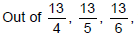, the first fraction is the biggest and the last one is the smallest.
What happens when if neither numerator nor denominator of the fractions is same. We know in this case, basic method is by LCM method, i.e. making the denominator same. But we don’t know that we can even make the numerator same. So we have to check that whose LCM is easy to find out, depending upon the value of the numerators or the denominators.

Let’s understand it with the help of an example.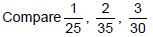Now we can see that finding out of the LCM of the 25, 35 and 30 is somewhat tedious. So we find out the LCM of the numerators, i.e. LCM of 1, 2 and 3. Which is 6. So our problem reduced to comparison of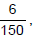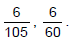Here we can check that being the numerator same, last fraction is the biggest.

Comparison of the fractions, when they are in certain fashion.

When relationship between each of the numerator and denominator is same, difference between them is same and with same sign. It has two cases.

1. If each numerator is smaller than its denominator by same value. In this situation the fraction having
bigger digits will be bigger in value and vice versa.
Let’s take one example: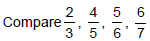Using above method we can easily compare all of them. Hence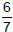is the biggest,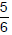is second biggest,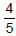is third biggest and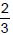is the smallest.

2. If each numerator is greater than its denominator by same value. In this situation the fraction having
bigger digits will be smaller and vice versa.
Let’s take one example:
Compare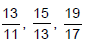Using above method we can easily say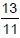is the biggest,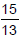is second biggest and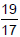is the
smallest.
Logical Comparison
This shortcut more depends upon our logic. The basic requirement to use this method is, we should be able to calculate the approximate value of the fraction.
Let’s take one example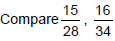• Now look at the first fraction, we see that denominator is 28 and half of the 28 is 14, 15 is greater than 14,
• Hence value of this fraction is slightly more thanSimilarly in second fraction, denominator is 34 and half of 34 is 17, 16 is less than 17, hence value of this fraction is slightly less than• Combining these two results we can judge that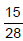is greater than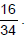## Percentage

Basic Shortcut

The most basic tip for the questions involving percentage is memorize the fractional equivalent of the

percentage. i.e.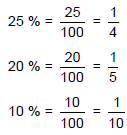Similarly, we can generate lot more.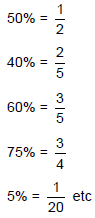This means when we have to calculate 25 % of 50, we can directly calculate one-fourth of 50, which is 12.5

Similarly, if we have to calculate 5 % of 400, we calculate one-twentieth (1/20) of the 400, which is 20.

Another shortcut

You may find it difficult to compute 18% of 25. Instead, try solving for 25% of 18.

25% is just half of half of any number. Half of 18 is 9, and half of 9 is 4.5.

Since 25% of 18 is 4.5, it is also true that 18% of 25 is 4.5.

This is something our teachers never taught us in school, and it would have helped a lot in everyday life -- A% of B = B% of A. Like multiplication, percentages conform to the commutative principle, which says the order of the terms does not matter. (Just as 2 x 3 = 3 x 2, it is also true that 40% of 5 = 5% of 40).

The document Vedic Mathematics: Solved Examples | Quantitative Aptitude (Quant) - CAT is a part of the CAT Course Quantitative Aptitude (Quant).
All you need of CAT at this link: CAT

## Quantitative Aptitude (Quant)

179 videos|154 docs|113 tests

## FAQs on Vedic Mathematics: Solved Examples - Quantitative Aptitude (Quant) - CAT

 1. What is Vedic Mathematics?Ans. Vedic Mathematics is a system of mathematics that originated in ancient India. It is based on 16 sutras (aphorisms) and 13 sub-sutras, which are short, catchy phrases that help in solving mathematical problems quickly and easily.
 2. What are some benefits of learning Vedic Mathematics?Ans. Some benefits of learning Vedic Mathematics include increased speed and accuracy in calculations, improved mental agility, and enhanced problem-solving skills. It can also help in reducing math anxiety and boosting confidence in math-related tasks.
 3. How can Vedic Mathematics be useful for competitive exams?Ans. Vedic Mathematics can be very useful for competitive exams as it provides quick and efficient methods for solving complex mathematical problems. It can help in saving time during the exam and can also improve accuracy in calculations, which is crucial for scoring high marks.
 4. What are some important topics covered in Vedic Mathematics?Ans. Some important topics covered in Vedic Mathematics include addition and subtraction, multiplication, division, fractions, decimals, and algebra. Specific techniques for each of these topics are provided in the Vedic Mathematics system.
 5. How can one learn Vedic Mathematics?Ans. Vedic Mathematics can be learned through various books, online courses, and workshops. It is important to find a reliable source that provides authentic information and guidance. Practicing regularly and applying the techniques in real-life situations can also help in mastering Vedic Mathematics.

## Quantitative Aptitude (Quant)

179 videos|154 docs|113 testsExplore Courses for CAT exam### How to Prepare for CAT

Read our guide to prepare for CAT which is created by Toppers & the best Teachers
Signup to see your scores go up within 7 days! Learn & Practice with 1000+ FREE Notes, Videos & Tests.
10M+ students study on EduRev
Track your progress, build streaks, highlight & save important lessons and more!
Related Searches

,

,

,

,

,

,

,

,

,

,

,

,

,

,

,

,

,

,

,

,

,

;